# Factorisation

## Exercise 6

#### Question 1

Find the remainder (without division) on dividing f(x) by (x - 2) where

(i) f(x) = 5x2 - 7x + 4

(ii) f(x) = 2x3 - 7x2 + 3

(i) By remainder theorem, on dividing f(x) by (x - a) , remainder = f(a)

∴ On dividing, f(x) = 5x2 - 7x + 4 by (x - 2)

Remainder = f(2)

$= 5(2)^2 - 7(2) + 4 \\[0.5em] = 5(4) - 14 + 4 \\[0.5em] = 20 - 10 \\[0.5em] = 10.$

Hence, the value of remainder is 10.

(ii) By remainder theorem, on dividing f(x) by (x - a) , remainder = f(a)

∴ On dividing, f(x) = 2x3 - 7x2 + 3 by (x - 2)

Remainder = f(2)

$= 2(2)^3 - 7(2)^2 + 3 \\[0.5em] = 2(8) - 28 + 3 \\[0.5em] = 16 - 28 + 3 \\[0.5em] = -9.$

Hence, the value of remainder is -9.

#### Question 2

Using remainder theorem, find the remainder on dividing f(x) by (x + 3) where

(i) f(x) = 2x2 - 5x + 1

(ii) f(x) = 3x3 + 7x2 - 5x + 1

(i) By remainder theorem, on dividing f(x) by (x - a) , remainder = f(a)

∴ On dividing, f(x) = 2x2 - 5x + 1 by (x + 3) or (x - (-3))

Remainder = f(-3)

$= 2(-3)^2 - 5(-3) + 1 \\[0.5em] = 2(9) + 15 + 1 \\[0.5em] = 18 + 16 \\[0.5em] = 34.$

Hence, the value of remainder is 34.

(ii) By remainder theorem, on dividing f(x) by (x - a) , remainder = f(a)

∴ On dividing, f(x) = 3x3 + 7x2 - 5x + 1 by (x + 3) or (x - (-3))

Remainder = f(-3)

$= 3(-3)^3 + 7(-3)^2 - 5(-3) + 1 \\[0.5em] = -81 + 63 + 15 + 1 \\[0.5em] = -2. \\[0.5em]$

Hence, the value of remainder is -2.

#### Question 3

Find the remainder (without division) on dividing f(x) by (2x + 1) where

(i) f(x) = 4x2 + 5x + 3

(ii) f(x) = 3x3 - 7x2 + 4x + 11

(i) By remainder theorem, on dividing f(x) by (x - a) , remainder = f(a)

∴ On dividing, f(x) = 4x2 + 5x + 3 by (2x + 1) or 2(x - (-$\dfrac{1}{2}$))

Remainder = f(-$\dfrac{1}{2}$)

$= 4\big(-\dfrac{1}{2}\big)^2 + 5\big(-\dfrac{1}{2}\big) + 3 \\[0.5em] = 4\big(\dfrac{1}{4}\big) -\dfrac{5}{2} + 3 \\[0.5em] = 1 - \dfrac{5}{2} + 3 \text{(On taking L.C.M.)} \\[0.5em] = \dfrac{2 - 5 + 6}{2} \\[0.5em] = \dfrac{3}{2} \\[0.5em] = 1\dfrac{1}{2}.$

Hence, the value of remainder is 1 $\dfrac{1}{2}$.

(ii) By remainder theorem, on dividing f(x) by (x - a) , remainder = f(a)

∴ On dividing, f(x) = 3x3 - 7x2 + 4x + 11 by (2x + 1) or 2(x - (-$\dfrac{1}{2}$))

Remainder = f$\big(-\dfrac{1}{2}\big)$

$= 3\big(-\dfrac{1}{2}\big)^3 - 7\big(-\dfrac{1}{2}\big)^2 + 4\big(-\dfrac{1}{2}\big) + 11 \\[0.5em] = 3\big(-\dfrac{1}{8}\big) - 7\big(\dfrac{1}{4}\big) - 2 + 11 \\[0.5em] = -\dfrac{3}{8} - \dfrac{7}{4} + 9 \\[0.5em] = \dfrac{-3 - 14 + 72}{8} \\[0.5em] = \dfrac{55}{8} \\[0.5em] = 6\dfrac{7}{8}$

Hence, the value of remainder is $6\dfrac{7}{8}$.

#### Question 4

Using remainder theorem, find the value of k if on dividing 2x3 + 3x2 - kx + 5 by (x - 2) leaves a remainder 7.

By remainder theorem, on dividing f(x) by (x - a) , remainder = f(a)

∴ On dividing, f(x) = 2x3 + 3x2 - kx + 5 by (x - 2)

Remainder = f(2)

Given, remainder = 7

$\therefore 2(2)^3 + 3(2)^2 - k(2) + 5 = 7 \\[0.5em] \Rightarrow 2(8) + 3(4) - 2k + 5 = 7 \\[0.5em] \Rightarrow 16 + 12 + 5 - 2k = 7 \\[0.5em] \Rightarrow 33 - 2k = 7 \\[0.5em] \Rightarrow 2k = 33 - 7 \\[0.5em] \Rightarrow 2k = 26 \\[0.5em] k = 13$

Hence, the value of k is 13.

#### Question 5

Using remainder theorem, find the value of a if the division of x3 + 5x2 - ax + 6 by (x - 1) leaves the remainder 2a.

By remainder theorem, on dividing f(x) by (x - a) , remainder = f(a)

∴ On dividing, f(x) = x3 + 5x2 - ax + 6 by (x - 1)

Remainder = f(1)

Given, remainder = 2a

$\therefore (1)^3 + 5(1)^2 - a(1) + 6 = 2a \\[0.5em] \Rightarrow 1 + 5 - a + 6 = 2a \\[0.5em] \Rightarrow 12 - a = 2a \\[0.5em] \Rightarrow 3a = 12 \\[0.5em] a = 4 \\[0.5em]$

Hence, the value of a is 4.

#### Question 6(i)

What number must be subtracted from 2x2 - 5x so that resulting polynomial leaves remainder 2 when divided by 2x + 1 ?

Let the number to be subtracted be a.

So, polynomial = 2x2 - 5x - a

By remainder theorem, on dividing f(x) by (x - b) , remainder = f(b)

∴ On dividing, f(x) = 2x2 - 5x - a by (2x + 1) or 2(x - $\big(-\dfrac{1}{2}\big)$)

Remainder = f$\big(-\dfrac{1}{2}\big)$

Given, remainder = 2

$\therefore 2\big(-\dfrac{1}{2}\big)^2 - 5\big(-\dfrac{1}{2}\big) - a = 2 \\[0.5em] \Rightarrow 2\big(\dfrac{1}{4}\big) + \dfrac{5}{2} - a = 2 \\[0.5em] \Rightarrow \dfrac{1}{2} + \dfrac{5}{2} - a = 2 \\[0.5em] \Rightarrow \dfrac{6}{2} - a = 2 \\[0.5em] \Rightarrow 3 - a = 2 \\[0.5em] \Rightarrow a = 3 - 2 \\[0.5em] a = 1.$

Hence, the value of a is 1.

#### Question 6(ii)

What number must be added to 2x3 - 7x2 + 2x so that the resulting polynomial leaves the remainder -2 when divided by 2x - 3 ?

Let the number to be added be a.

So, polynomial = 2x3 - 7x2 + 2x + a

By remainder theorem, on dividing f(x) by (x - b) , remainder = f(b)

∴ On dividing, f(x) = 2x3 - 7x2 + 2x + a by (2x - 3) or 2(x - $\dfrac{3}{2}$))

Remainder = f$\big(\dfrac{3}{2}\big)$

Given, remainder = -2

$\therefore 2\big(\dfrac{3}{2}\big)^3 - 7\big(\dfrac{3}{2}\big)^2 + 2\big(\dfrac{3}{2}\big) + a = -2 \\[0.5em] \Rightarrow 2\big(\dfrac{27}{8}\big) - 7\big(\dfrac{9}{4}\big) + 3 + a = -2 \\[0.5em] \Rightarrow \dfrac{27}{4} - \dfrac{63}{4} + 3 + a = -2 \\[0.5em] \Rightarrow \dfrac{27 - 63 + 12}{4} + a = -2 \\[0.5em] \Rightarrow -\dfrac{24}{4} + a = -2 \\[0.5em] \Rightarrow a = 6 - 2 \\[0.5em] a = 4.$

Hence, the value of a is 4.

#### Question 7(i)

When divided by x - 3 the polynomials x3 - px2 + x + 6 and 2x3 - x2 - (p + 3)x - 6 leave the same remainder . Find the value of 'p'.

By remainder theorem, on dividing f(x) by (x - b), remainder = f(b)

∴ On dividing, f(x) = x3 - px2 + x + 6 by (x - 3)

Remainder = 33 - p(32) + 3 + 6 = 27 - 9p + 9 = 36 - 9p.

∴ On dividing, f(x) = 2x3 - x2 - (p + 3)x - 6 by (x - 3)

Remainder = 2(3)3 - 32 - (p + 3)(3) - 6 = 2(27) - 9 - 3p - 9 - 6 = 54 - 9 - 3p - 9 - 6 = 30 - 3p

According to question,

$\Rightarrow 36 - 9p = 30 - 3p \\[0.5em] \Rightarrow 36 - 30 = 9p - 3p \\[0.5em] \Rightarrow 6 = 6p \\[0.5em] \Rightarrow p = 1$

Hence, the value of p is 1.

#### Question 7(ii)

Find 'a' if the two polynomials ax3 + 3x2 - 9 and 2x3 + 4x + a, leaves the same remainder when divided by x + 3.

By remainder theorem, on dividing f(x) by (x - b), remainder = f(b)

∴ On dividing, f(x) = ax3 + 3x2 - 9 by (x + 3) or (x - (-3))

Remainder = f(-3) = a(-3)3 + 3(-3)2 - 9 = -27a + 27 - 9 = 18 - 27a

∴ On dividing, f(x) = f(-3) = 2x3 + 4x + a by (x + 3) or (x - (-3))

Remainder = 2(-3)3 + 4(-3) + a = -54 - 12 + a = a - 66

According to question,

$\Rightarrow 18 - 27a = a - 66 \\[0.5em] \Rightarrow a + 27a = 66 + 18 \\[0.5em] \Rightarrow 28a = 84 \\[0.5em] \Rightarrow a = \dfrac{84}{28} \\[0.5em] \Rightarrow a = 3.$

Hence, the value of p is 3.

#### Question 7(iii)

The polynomials ax3 + 3x2 - 3 and 2x3 - 5x + a when divided by x - 4 leave the remainder r1 and r2 respectively. If 2r1 = r2, then find the value of a.

By remainder theorem, on dividing f(x) by (x - b), remainder = f(b)

∴ On dividing, f(x) = ax3 + 3x2 - 3 by (x - 4)

Remainder = f(4) = a(4)3 + 3(4)2 - 3 = 64a + 45

∴ On dividing, f(x) = 2x3 - 5x + a by (x - 4)

Remainder = f(4) = 2(4)3 - 5(4) + a = 128 - 20 + a = 108 + a

According to question,

r1 = 64a + 45

r2 = 108 + a

2r1 = r2

$\therefore 2(64a + 45) = 108 + a \\[0.5em] \Rightarrow 128a + 90 = 108 + a \\[0.5em] \Rightarrow 128a - a = 108 - 90 \\[0.5em] \Rightarrow 127a = 18 \\[0.5em] \Rightarrow a = \dfrac{18}{127}.$

#### Question 8

Using the remainder theorem, find the remainders obtained when x3 + (kx + 8)x + k is divided by x + 1 and x - 2. Hence, find k if the sum of two remainders is 1.

By remainder theorem, on dividing f(x) by (x - b), remainder = f(b)

∴ On dividing, f(x) = x3 + (kx + 8)x + k by x + 1 or (x - (-1))

Remainder = r1 = f(-1) = -13 + ((-1)k + 8)(-1) + k
= -1 + (8 - k)(-1) + k
= -1 - 8 + k + k
= 2k - 9

∴ On dividing, f(x) = x3 + (kx + 8)x + k by x - 2

Remainder = r2 = (2)3 + (k(2) + 8)2 + k
= 8 + 4k + 16 + k
= 5k + 24

Given, sum of two remainders = 1

∴ r1 + r2 = 1

$\Rightarrow 2k - 9 + 5k + 24 = 1 \\[0.5em] \Rightarrow 7k + 15 = 1 \\[0.5em] \Rightarrow 7k = 1 - 15 \\[0.5em] \Rightarrow 7k = -14 \\[0.5em] \Rightarrow k = -\dfrac{14}{7} \\[0.5em] k = -2.$

The first remainder is 2k - 9 and the second remainder is 5k + 24 and the value of k is -2.

#### Question 9

By factor theorem, show that (x + 3) and (2x - 1) are the factors of 2x2 + 5x - 3.

By factor theorem, (x - a) is a factor of f(x), if f(a) = 0.

f(x) = 2x2 + 5x - 3

(x + 3) = (x - (-3)) is a factor of f(x), if f(-3) = 0

f(-3) = 2(-3)2 + 5(-3) - 3
= 2(9) - 15 - 3
= 18 - 18 = 0

2x - 1 = 2(x - $\dfrac{1}{2}$) is a factor of f(x), if f($\dfrac{1}{2}$) = 0

$f\big(\dfrac{1}{2}\big) = 2\big(\dfrac{1}{2}\big)^2 + 5\big(\dfrac{1}{2}\big) - 3 \\[0.5em] = 2\big(\dfrac{1}{4}\big) + \dfrac{5}{2} - 3 \\[0.5em] = \dfrac{1}{2} + \dfrac{5}{2} - 3 \\[0.5em] = 3 - 3 = 0$

Since, f(-3) and f$\big(\dfrac{1}{2}\big)$ = 0 , hence, (x - 3) and (2x - 1) are factors of 2x2 + 5x - 3.

#### Question 10

Without actual division, prove that x4 + 2x3 - 2x2 + 2x - 3 is exactly divisible by x2 + 2x - 3.

Let, f(x) = x4 + 2x3 - 2x2 + 2x - 3

g(x) = x2 + 2x - 3
= x2 + 3x - x - 3
= x(x + 3) - 1(x + 3)
= (x - 1)(x + 3)

$\Rightarrow$ (x - 1) and (x + 3) are factors of g(x).

In order to prove that f(x) is exactly divisible by g(x), it is sufficient to prove that x - 1 and x + 3 are factors of f(x) i.e. it is sufficient to show that f(1) = 0 and f(-3) = 0.

Now,
f(1) = (1)4 + 2(1)3 - 2(1)2 + 2(1) - 3

= 1 + 2 - 2 + 2 - 3 = 0

f(-3) = (-3)4 + 2(-3)3 - 2(-3)2 + 2(-3) - 3

= 81 - 54 - 18 - 6 - 3 = 0

∴ f(x) is divisible by (x - 1) and (x + 3)

Hence, f(x) is exactly divisible by g(x).

#### Question 11

Show that (x - 2) is a factor 3x2 - x - 10. Hence, factorize 3x2 - x - 10.

By factor theorem, (x - a) is a factor of f(x), if f(a) = 0.

f(x) = 3x2 - x - 10

(x - 2) is a factor of f(x), if f(2) = 0

f(2) = 3(2)2 - 2 - 10

= 12 - 12 = 0

Hence, x - 2 is a factor of 3x2 - x - 10.

Now, factorizing $3x^2 - x - 10$,

$\Rightarrow 3x^2 - 6x + 5x - 10 \\[0.5em] \Rightarrow 3x(x - 2) + 5(x - 2) \\[0.5em] \Rightarrow (3x + 5)(x - 2)$

Hence, 3x2 - x - 10 = (x - 2)(3x + 5).

#### Question 12

Using factor theorem, show that (x - 2) is a factor of x3 + x2 - 4x - 4. Hence, factorise the polynomial completely.

By factor theorem, (x - a) is a factor of f(x), if f(a) = 0.

f(x) = x3 + x2 - 4x - 4

(x - 2) is a factor of f(x), if f(2) = 0

$f(2) = (2)^3 + (2)^2 - 4(2) - 4 \\[0.5em] = 8 + 4 - 8 - 4 \\[0.5em] = 0$

Hence, (x - 2) is a factor of x3 + x2 - 4x - 4.

Now, factorizing x3 + x2 - 4x - 4,

$\Rightarrow x^2(x + 1) - 4(x + 1) \\[0.5em] \Rightarrow (x^2 - 4)(x + 1) \\[0.5em] \Rightarrow (x - 2)(x + 2)(x + 1)$

Hence, x3 + x2 - 4x - 4 = (x - 2)(x + 1)(x + 2).

#### Question 13

Show that 2x + 7 is a factor of 2x3 + 5x2 - 11x - 14. Hence, factorise the given expression completely, using the factor theorem.

By factor theorem, (x - a) is a factor of f(x), if f(a) = 0.

f(x) = 2x3 + 5x2 - 11x - 14

(2x + 7) or $2(x - \big(-\dfrac{7}{2}\big))$ is a factor of f(x), if $f\big(-\dfrac{7}{2}\big)$ = 0

$f\big(-\dfrac{7}{2}\big) = 2\big(-\dfrac{7}{2}\big)^3 + 5\big(-\dfrac{7}{2}\big)^2 - 11\big(-\dfrac{7}{2}\big) -14 \\[0.5em] = 2\big(-\dfrac{343}{8}\big) + 5\big(\dfrac{49}{4}\big) + \big(\dfrac{77}{2}\big) - 14 \\[0.5em] = \big(-\dfrac{343}{4}\big) + \big(\dfrac{245}{4}\big) + \big(\dfrac{77}{2}\big) - 14 \\[0.5em] = \big(\dfrac{-343 + 245 + 154 - 56}{4}\big) \text{(On } \text{taking } \text{L.C.M)} \\[0.5em] = \big(\dfrac{-399 + 399}{4}\big) \\[0.5em] = 0$

Hence, (2x + 7) is the factor of f(x).

On dividing f(x) by (2x + 7),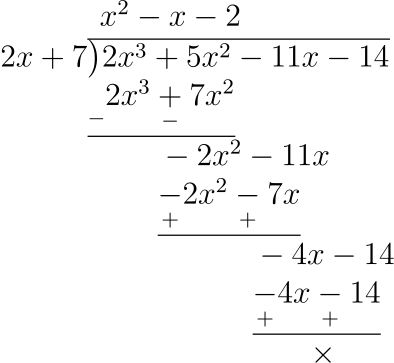we get, x2 - x - 2 as the quotient and remainder = 0.

$\therefore 2x^3 + 5x^2 - 11x - 14 = (2x + 7)(x^2 - x - 2) \\[0.5em] = (2x + 7)(x^2 - 2x + x - 2) \\[0.5em] = (2x + 7)(x(x - 2) + 1(x - 2)) \\[0.5em] = (2x + 7)(x + 1)(x - 2)$

Hence, 2x3 + 5x2 - 11x - 14 = (2x + 7)(x + 1)(x - 2)

#### Question 14

Use factor theorem to factorise the following polynomials completely :

(i) x3 + 2x2 - 5x - 6

(ii) x3 - 13x - 12

(i) f(x) = x3 + 2x2 - 5x - 6

Putting, x = -1 in f(x)

$f(-1) = (-1)^3 + 2(-1)^2 - 5(-1) - 6 \\[0.5em] = -1 + 2 + 5 - 6 \\[0.5em] = 7 - 7 \\[0.5em] = 0$

Since, f(-1) = 0, hence (x + 1) is factor of f(x) by factor theorem.

Now, dividing f(x) by (x + 1),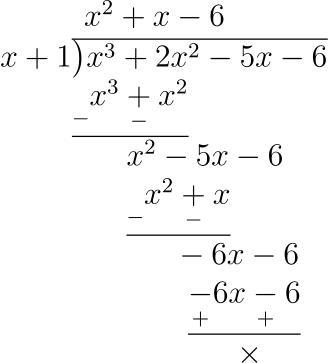we get x2 + x - 6 as the quotient and remainder = 0.

$\therefore x^3 + 2x^2 - 5x - 6 = (x + 1)(x^2 + x - 6) \\[0.5em] = (x + 1)(x^2 + 3x - 2x - 6) \\[0.5em] = (x + 1)(x(x + 3) - 2(x + 3)) \\[0.5em] = (x + 1)(x - 2)(x + 3)$

Hence, x3 + 2x2 - 5x - 6 = (x + 1)(x - 2)(x + 3).

(ii) Let f(x) = x3 - 13x - 12

Putting, x = 4 in f(x)

$f(4) = (4)^3 - 13(4) - 12 \\[0.5em] = 64 - 52 - 12 \\[0.5em] = 64 - 64 \\[0.5em] = 0$

Since, f(4) = 0, hence (x - 4) is factor of f(x) by factor theorem.

Now, dividing f(x) by (x - 4),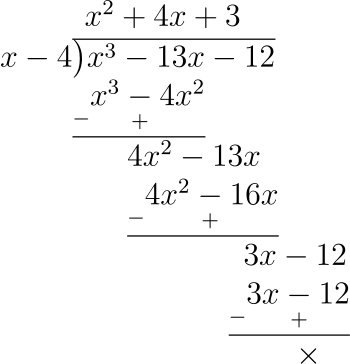we get x2 + 4x + 3 as quotient and remainder = 0.

$\therefore x^3 - 13x - 12 = (x - 4)(x^2 + 4x + 3) \\[0.5em] = (x - 4)(x^2 + 3x + x + 3) \\[0.5em] = (x - 4)(x(x + 3) + 1(x + 3)) \\[0.5em] = (x - 4)(x + 1)(x + 3)$

Hence, x3 - 13x - 12 = (x - 4)(x + 1)(x + 3).

#### Question 15

Use Remainder Theorem to factorise the following polynomials completely :

(i) 2x3 + x2 - 13x + 6

(ii) 3x3 + 2x2 - 19x + 6

(iii) 2x3 + 3x2 - 9x - 10

(iv) x3 + 10x2 - 37x + 26

(i) Let f(x) = 2x3 + x2 - 13x + 6

Putting, x = 2 in f(x)

$f(2) = 2(2)^3 + 2^2 - 13(2) + 6 \\[0.5em] = 16 + 4 - 26 + 6 \\[0.5em] = 0$

Since, f(2) = 0 , (x - 2) is factor of f(x) by factor theorem.
Dividing, f(x) by (x - 2),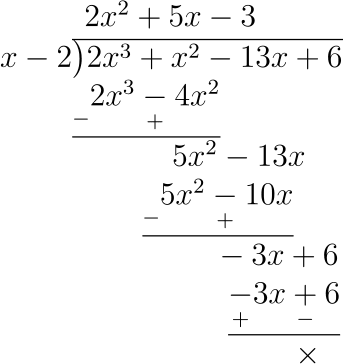we get, 2x2 + 5x - 3 as quotient and remainder = 0.

$\therefore 2x^3 + x^2 - 13x + 6 = (x - 2)(2x^2 + 5x - 3) \\[0.5em] = (x - 2)(2x^2 + 6x - x - 3) \\[0.5em] = (x - 2)(2x(x + 3) - 1(x + 3)) \\[0.5em] = (x - 2)(2x - 1)(x + 3)$

Hence, 2x3 + x2 - 13x + 6 = (x - 2)(2x - 1)(x + 3).

(ii) Let f(x) = 3x3 + 2x2 - 19x + 6

Putting, x = 2 in f(x)

$f(2) = 3(2)^3 + 2(2)^2 - 19(2) + 6 \\[0.5em] = 24 + 8 - 38 + 6 \\[0.5em] = 38 - 38 \\[0.5em] = 0$

Since, f(2) = 0, (x - 2) is factor of f(x) by factor theorem.

Dividing, f(x) by (x - 2),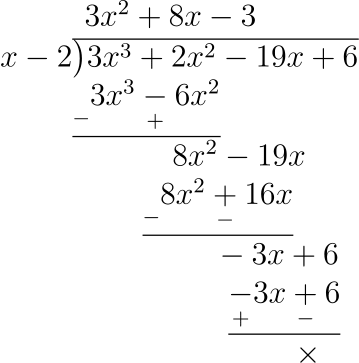we get, 3x2 + 8x - 3 as quotient and remainder = 0.

$\therefore 3x^3 + 2x^2 - 19x + 6 = (x - 2)(3x^2 + 8x - 3) \\[0.5em] = (x - 2)(3x^2 + 9x - x - 3) \\[0.5em] = (x - 2)(3x(x + 3) - 1(x + 3)) \\[0.5em] = (x - 2)(3x - 1)(x + 3)$

Hence, 3x3 + 2x2 - 19x + 6 = (x - 2)(3x - 1)(x + 3).

(iii) Let f(x) = 2x3 + 3x2 - 9x - 10

Putting, x = 2 in f(x)

$f(2) = 2(2)^3 + 3(2)^2 - 9(2) - 10 \\[0.5em] = 16 + 12 - 18 - 10 \\[0.5em] = 28 - 28 \\[0.5em] = 0$

Since, f(2) = 0, (x - 2) is factor of f(x) by factor theorem.

Dividing, f(x) by (x - 2),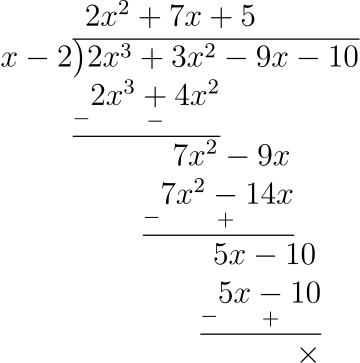we get, 2x2 + 7x + 5 as quotient and remainder = 0.

$\therefore 2x^3 + 3x^2 - 9x - 10 = (x - 2)(2x^2 + 7x + 5) \\[0.5em] = (x - 2)(2x^2 + 5x + 2x + 5) \\[0.5em] = (x - 2)(x(2x + 5) + 1(2x + 5)) \\[0.5em] = (x - 2)(x + 1)(2x + 5)$

Hence, 2x3 + 3x2 - 9x - 10 = (x - 2)(x + 1)(2x + 5).

(iv) Let f(x) = x3 + 10x2 - 37x + 26

Putting, x = 1 in f(x)

$f(1) = (1)^3 + 10(1)^2 - 37(1) + 26 \\[0.5em] = 1 + 10 - 37 + 26 \\[0.5em] = 37 - 37 \\[0.5em] = 0$

Since, f(1) = 0 , (x - 1) is factor of f(x) by factor theorem.

Dividing, f(x) by (x - 1),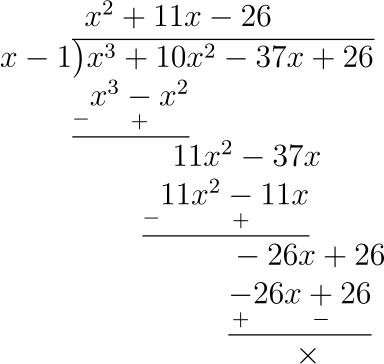we get, x2 + 11x - 26 as quotient and remainder = 0.

$\therefore x^3 + 10x^2 - 37x + 26 = (x - 1)(x^2 + 11x - 26) \\[0.5em] = (x - 1)(x^2 + 13x - 2x - 26) \\[0.5em] = (x - 1)(x(x + 13) - 2(x + 13)) \\[0.5em] = (x - 1)(x - 2)(x + 13)$

Hence, x3 + 10x2 - 37x + 26 = (x - 1)(x - 2)(x + 13).

#### Question 16

If (2x + 1) is a factor of 6x3 + 5x2 + ax - 2, find the value of a.

f(x) = 6x3 + 5x2 + ax - 2

If, (2x + 1) or 2(x - (-$\dfrac{1}{2}$)) is a factor of f(x) then f(-$\dfrac{1}{2}$) = 0

$\therefore 6\big(-\dfrac{1}{2}\big)^3 + 5\big(-\dfrac{1}{2}\big)^2 + a(-\dfrac{1}{2}) - 2 = 0 \\[0.5em] \Rightarrow -\dfrac{3}{4} + \dfrac{5}{4} - \dfrac{a}{2} - 2 = 0 \\[0.5em] \Rightarrow \dfrac{-3 + 5 - 2a - 8}{4} = 0 \text{(On taking L.C.M.)} \\[0.5em] \Rightarrow \dfrac{-6 - 2a}{4} = 0 \\[0.5em] \Rightarrow -6 - 2a = 0 \text{(On cross multiplying)} \\[0.5em] \Rightarrow 2a = -6 \\[0.5em] a = -3.$

Hence, the value of a is -3.

#### Question 17

If (3x - 2) is a factor of 3x3 - kx2 + 21x - 10, find the value of k.

f(x) = 3x3 - kx2 + 21x - 10

If, (3x - 2) or $3(x - \big(\dfrac{2}{3}\big))$ is a factor of f(x) then f($\dfrac{2}{3}$) = 0

$\therefore 3\big(\dfrac{2}{3}\big)^3 - k\big(\dfrac{2}{3}\big)^2 + 21\big(\dfrac{2}{3}\big) - 10 = 0 \\[0.5em] \Rightarrow 3\big(\dfrac{8}{27}\big) - k\big(\dfrac{4}{9}\big) + 14 - 10 = 0 \\[0.5em] \Rightarrow \dfrac{8}{9} - \dfrac{4k}{9} + 4 = 0 \\[0.5em] \Rightarrow \dfrac{8 - 4k + 36}{9} = 0 \\[0.5em] \Rightarrow 44 - 4k = 0 \\[0.5em] \Rightarrow 4k = 44 \\[0.5em] k = 11.$

Hence, the value of k is 11.

#### Question 18

If (x - 2) is a factor of 2x3 - x2 - px - 2, then

(i) Find the value of p.

(ii) with this value of p, factorise the above expression completely.

(i) f(x) = 2x3 - x2 - px - 2

If, (x - 2) is a factor of f(x), then f(2) = 0

$\therefore 2(2)^3 - (2)^2 - 2p - 2 = 0 \\[0.5em] \Rightarrow 16 - 4 - 2 - 2p = 0 \\[0.5em] \Rightarrow 10 - 2p = 0 \\[0.5em] \Rightarrow 2p = 10 \\[0.5em] p = 5.$

Hence, the value of p is 5.

(ii) Putting value of p = 5 in f(x),

f(x) = 2x3 - x2 - 5x - 2

Since, (x - 2) is a factor of f(x), dividing f(x) by (x - 2),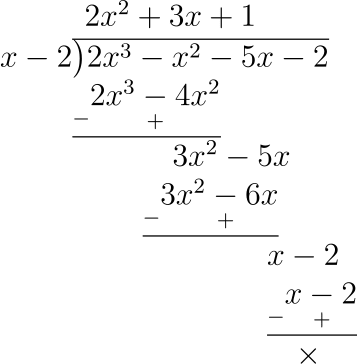we get, 2x2 + 3x + 1 as quotient and remainder = 0.

$\therefore 2x^3 - x^2 - 5x - 2 = (x - 2)(2x^2 + 3x + 1) \\[0.5em] = (x - 2)(2x^2 + 2x + x + 1) \\[0.5em] = (x - 2)(2x(x + 1) + 1(x + 1)) \\[0.5em] = (x - 2)(2x + 1)(x + 1)$

Hence, 2x3 - x2 - 5x - 2 = (x - 2)(2x + 1)(x + 1).

#### Question 19

What number should be subtracted from 2x3 - 5x2 + 5x so that the resulting polynomial has 2x - 3 as a factor?

Let the number to be subtracted be a.

f(x) = 2x3 - 5x2 + 5x - a

If, (2x - 3) or 2(x - ($\dfrac{3}{2}$)) is a factor of f(x) then f($\dfrac{3}{2}$) = 0, by factor theorem

$\therefore 2\big(\dfrac{3}{2}\big)^3 - 5\big(\dfrac{3}{2}\big)^2 + 5(\dfrac{3}{2}) - a = 0 \\[0.5em] \Rightarrow 2\big(\dfrac{27}{8}\big) - 5\big(\dfrac{9}{4}\big) + \dfrac{15}{2} - a = 0 \\[0.5em] \Rightarrow \dfrac{27}{4} - \dfrac{45}{4} + \dfrac{15}{2} - a = 0 \\[0.5em] \Rightarrow \dfrac{27 - 45 + 30 - 4a}{4} = 0 \\[0.5em] \Rightarrow 12 - 4a = 0 \\[0.5em] \Rightarrow 4a = 12 \\[0.5em] a = 3.$

Hence, the number to be subtracted is 3.

#### Question 20(i)

Find the value of the constants a and b, if (x - 2) and (x + 3) are both factors of the expression x3 + ax2 + bx - 12.

f(x) = x3 + ax2 + bx - 12

If (x - 2) and (x + 3) or (x - (-3)) are factors of f(x) then, f(2) and f(-3) = 0.

$\therefore f(2) = 2^3 + a(2)^2 + 2b - 12 = 0 \\[0.5em] \Rightarrow 8 + 4a + 2b - 12 = 0 \\[0.5em] \Rightarrow 4a + 2b - 4 = 0 \\[0.5em] \Rightarrow 4a + 2b = 4 \\[0.5em] \Rightarrow 2a + b = 2 \text{ (On dividing equation by 2) } \\[0.5em] b = 2 - 2a \text{ \space (Equation 1)}$

$\therefore f(-3) = (-3)^3 + a(-3)^2 + (-3)b - 12 = 0 \\[0.5em] \Rightarrow -27 + 9a - 3b - 12 = 0 \\[0.5em] \Rightarrow 9a - 3b - 39 = 0 \\[0.5em] \text{(Putting value of b = 2 - 2a from equation 1 )} \\[0.5em] \Rightarrow 9a - 3(2 - 2a) - 39 = 0 \\[0.5em] \Rightarrow 9a - 6 + 6a - 39 = 0 \\[0.5em] \Rightarrow 15a - 45 = 0 \\[0.5em] \Rightarrow 15a = 45 \\[0.5em] \Rightarrow a = 3 \\[0.5em] \therefore b = 2 - 2a = 2 - 6 = -4$

Hence, the value of a is 3 and that of b is -4.

#### Question 20(ii)

If (x + 2) and (x + 3) are factors of x3 + ax + b, find the values of a and b.

f(x) = x3 + ax + b

If (x + 2) or (x - (-2)) and (x + 3) or (x - (-3)) are factors of f(x) then, f(-2) and f(-3) = 0.

$\therefore f(-2) = (-2)^3 + (-2)a + b = 0 \\[0.5em] \Rightarrow -8 - 2a + b = 0 \\[0.5em] \Rightarrow b = 2a + 8 \text{ \space (Equation 1)}$

$\therefore f(-3) = (-3)^3 + (-3)a + b = 0 \\[0.5em] \Rightarrow -27 - 3a + b = 0 \\[0.5em] \text{(Putting value of b = 2a + 8 from equation 1 )} \\[0.5em] \Rightarrow -27 - 3a + 2a + 8 = 0 \\[0.5em] \Rightarrow -27 - a + 8 = 0 \\[0.5em] \Rightarrow -a - 19 = 0 \\[0.5em] \Rightarrow a = -19 \\[0.5em] \therefore b = 2a + 8 = -38 + 8 = -30$

Hence, the value of a is -19 and that of b is -30.

#### Question 21

If (x + 2) and (x - 3) are the factors of x3 + ax + b, find the values of a and b. With these values of a and b, factorise the given expression.

f(x) = x3 + ax + b

If (x + 2) or (x - (-2)) and (x - 3) are factors of f(x) then, f(-2) and f(3) = 0.

$\therefore f(-2) = (-2)^3 + (-2)a + b = 0 \\[0.5em] \Rightarrow -8 - 2a + b = 0 \\[0.5em] \Rightarrow b = 2a + 8 \text{ \space (Equation 1)}$

$\therefore f(3) = (3)^3 + (3)a + b = 0 \\[0.5em] \Rightarrow 27 + 3a + b = 0 \\[0.5em] \text{(Putting value of b = 2a + 8 from equation 1 )} \\[0.5em] \Rightarrow 27 + 3a + 2a + 8 = 0 \\[0.5em] \Rightarrow 35 + 5a = 0 \\[0.5em] \Rightarrow 5a = -35 \\[0.5em] \Rightarrow a = -7 \\[0.5em] \therefore b = 2a + 8 = -14 + 8 = -6$

Putting the values of a and b in f(x) we get,

f(x) = x3 - 7x - 6

Since, (x + 2) and (x - 3) are factors of f(x) hence, (x + 2)(x - 3) = (x2 - x - 6) is also the factor.

On dividing f(x) by x2 - x - 6,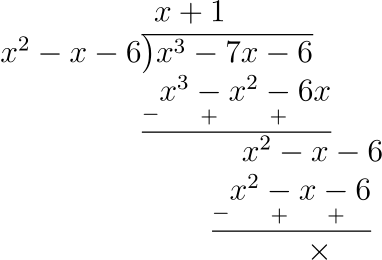we get, (x + 1) as quotient and remainder = 0.

$\therefore x^3 - 7x - 6 = (x^2 - x - 6)(x + 1) \\[0.5em] = (x^2- 3x + 2x - 6)(x + 1) \\[0.5em] = (x(x - 3) + 2(x - 3))(x + 1) \\[0.5em] = (x + 2)(x - 3)(x + 1)$

Hence, the value of a = -7 and b = -6;
x3 - 7x - 6 = (x + 2)(x - 3)(x + 1).

#### Question 22

(x - 2) is a factor of the expression x3 + ax2 + bx + 6. When this expression is divided by (x - 3), it leaves the remainder 3. Find the values of a and b.

Let f(x) = x3 + ax2 + bx + 6

Given, (x - 2) is factor of f(x), hence, f(2) = 0 by factor's theorem

$\therefore 2^3 + a(2)^2 + b(2) + 6 = 0 \\[0.5em] \Rightarrow 8 + 4a + 2b + 6 = 0 \\[0.5em] \Rightarrow 4a + 2b + 14 = 0 \\[0.5em] \Rightarrow 2a + b + 7 = 0 \\[0.5em] b = -7 - 2a \text{ (Equation 1) }$

Given, on dividing f(x) by (x - 3) remainder left is 3

By remainder theorem, remainder = f(3)

$\therefore 3^3 + a(3)^2 + b(3) + 6 = 3 \\[0.5em] \Rightarrow 27 + 9a + 3b + 6 = 3 \\[0.5em] \Rightarrow 33 + 9a + 3b = 3 \\[0.5em] \Rightarrow 11 + 3a + b = 1 \text{ (On dividing equation by 3)} \\[0.5em] \text{ Putting value of b from equation 1 } \\[0.5em] \Rightarrow 11 + 3a - 7 - 2a = 1 \\[0.5em] \Rightarrow a + 4 = 1 \\[0.5em] \Rightarrow a = -3 \\[0.5em] \text{ and } b = -7 - 2a = -7 - 2(-3) = -7 + 6 = -1$

Hence, the value of a = -3 and b = -1.

#### Question 23

If (x - 2) is a factor of the expression 2x3 + ax2 + bx - 14 and when the expression is divided by (x - 3), it leaves a remainder 52, find the values of a and b.

Let f(x) = 2x3 + ax2 + bx - 14

Given, (x - 2) is factor of f(x), hence, f(2) = 0 by factor's theorem

$\therefore 2(2)^3 + a(2)^2 + b(2) - 14 = 0 \\[0.5em] \Rightarrow 16 + 4a + 2b - 14 = 0 \\[0.5em] \Rightarrow 2 + 4a + 2b = 0 \\[0.5em] \Rightarrow 2a + b + 1 = 0 \text{ (On dividing equation by 2) }\\[0.5em] \Rightarrow b = -1 - 2a \text{ (Equation 1) }$

Given, on dividing f(x) by (x - 3) remainder left is 52

By remainder theorem, remainder = f(3)

$\therefore 2(3)^3 + a(3)^2 + b(3) - 14 = 52 \\[0.5em] \Rightarrow 54 + 9a + 3b - 14 = 52 \\[0.5em] \Rightarrow 9a + 3b + 40 = 52 \\[0.5em] \Rightarrow 9a + 3b = 12 \\[0.5em] \Rightarrow 3a + b = 4 \\[0.5em] \text{ Putting value of b from equation 1 } \\[0.5em] \Rightarrow 3a - 1 - 2a = 4 \\[0.5em] \Rightarrow a = 5 \\[0.5em] \text{ and } b = -1 - 2a = -1 - 10 = -11$

Hence, the value of a = 5 and b = -11.

#### Question 24

If ax3 + 3x2 + bx - 3 has a factor (2x + 3) and leaves remainder -3 when divided by (x + 2), find the values of a and b. With these values of a and b, factorise the given expression.

Let f(x) = ax3 + 3x2 + bx - 3

Given, (2x + 3) or $2(x - \big(-\dfrac{3}{2}\big))$ is factor of f(x), hence, f$\big(-\dfrac{3}{2}\big)$ = 0 by factor's theorem

$\therefore a\big(-\dfrac{3}{2}\big)^3 + 3\big(-\dfrac{3}{2}\big)^2 + b\big(-\dfrac{3}{2}\big) - 3 = 0 \\[0.5em] \Rightarrow -\dfrac{27a}{8} + \dfrac{27}{4} - \dfrac{3b}{2} - 3 = 0 \\[0.5em] \Rightarrow \big(\dfrac{-27a + 54 - 12b - 24}{8}\big) = 0 \\[0.5em] \Rightarrow -27a - 12b + 30 = 0 \text{ (On cross multiplication) } \\[0.5em] \Rightarrow -9a - 4b + 10 = 0 \text{ (On dividing the equation by 3) }\\[0.5em] \Rightarrow 4b + 9a = 10 \text{( Equation 1)}$

Given, on dividing f(x) by (x + 2) remainder left is -3

By remainder theorem, remainder = f(-2)

$\therefore a(-2)^3 + 3(-2)^2 + b(-2) - 3 = -3 \\[0.5em] \Rightarrow -8a + 12 - 2b - 3 = -3 \\[0.5em] \Rightarrow -8a - 2b + 9 = -3 \\[0.5em] \Rightarrow -8a - 2b = -12 \\[0.5em] \Rightarrow -4a - b = -6 \text{ (On dividing equation by -2) }\\[0.5em] \Rightarrow 4a + b = 6 \\[0.5em] \Rightarrow 16a + 4b = 24 \text{ (Multiplying equation by 4) }\\[0.5em] \text{ (Subtracting above equation from equation 1) } \\[0.5em] \Rightarrow 4b + 9a - 16a - 4b = 10 - 24 \\[0.5em] \Rightarrow -7a = -14 \\[0.5em] \Rightarrow a = 2 \\[0.5em] \text{and } b = 6 - 4a = 6 - 8 = -2.$

Putting value of a and b in f(x) we get,

$f(x) = 2x^3 + 3x^2 - 2x - 3 \\[0.5em] = x^2(2x + 3) -1(2x + 3) \\[0.5em] = (x^2 - 1)(2x + 3) \\[0.5em] = ((x)^2 - (1)^2)(2x + 3) \\[0.5em] = (x - 1)(x + 1)(2x + 3)$

Hence, the value of a = 2 and b = -2 ; 2x3 + 3x2 - 2x - 3 = (x - 1)(x + 1)(2x + 3).

#### Question 25

Given f(x) = ax2 + bx + 2 and g(x) = bx2 + ax + 1. If (x - 2) is a factor of f(x) but leaves the remainder -15 when it divides g(x), find the values of a and b. With these values of a and b, factorise the expression

f(x) + g(x) + 4x2 + 7x

f(x) = ax2 + bx + 2

Given, (x - 2) is a factor of f(x) hence, by factor theorem f(2) = 0

$\therefore a(2)^2 + b(2) + 2 = 0 \\[0.5em] \Rightarrow 4a + 2b + 2 = 0 \\[0.5em] \Rightarrow 2a + b + 1 = 0 \text{ (On dividing equation by 2) } \\[0.5em] b = -1 - 2a$

g(x) = bx2 + ax + 1

Given, on dividing g(x) by (x - 2), remainder = -15 and by remainder theorem, remainder = g(2)

$\therefore g(2) = -15 \\[0.5em] \Rightarrow b(2)^2 + a(2) + 1 = -15 \\[0.5em] \Rightarrow 4b + 2a = -15 - 1 \\[0.5em] \Rightarrow 4b + 2a = -16 \\[0.5em] \Rightarrow 2b + a = -8 \text{ (On dividing equation by 2) } \\[0.5em] \text{ Putting value of } b = -1 - 2a \text{ from equation 1} \\[0.5em] \Rightarrow 2(-1 - 2a) + a = -8 \\[0.5em] \Rightarrow -2 - 4a + a = -8 \\[0.5em] \Rightarrow -3a = -8 + 2 \\[0.5em] \Rightarrow a = \dfrac{-6}{-3} \\[0.5em] \Rightarrow a = 2 \\[0.5em] \text{ and } b = -1 - 2a = -1 - 4 = -5.$

Putting value of a = 2 and b = -5 in f(x) + g(x) + 4x2 + 7x we get,

$(2x^2 - 5x + 2) + (-5x^2 + 2x + 1) + 4x^2 + 7x \\[0.5em] = 2x^2 - 5x^2 + 4x^2 - 5x + 2x + 7x + 2 + 1 \\[0.5em] = x^2 + 4x + 3 \\[0.5em] = x^2 + 3x + x + 3 \\[0.5em] = x(x + 3) + 1(x + 3) \\[0.5em] = (x + 1)(x + 3)$

Hence, the value of a = 2 and b = -5;
f(x) + g(x) + 4x2 + 7x = (x + 1)(x + 3).

## Multiple Choice Questions

Choose the correct answer from the given four options(1 to 4):

#### Question 1

When 2x3 - x2 - 3x + 5 is divided by 2x + 1, then the remainder is

1. 6
2. -6
3. -3
4. 0

By remainder theorem, on dividing f(x) by (x - a), the remainder left is f(a).

f(x) = 2x3 - x2 - 3x + 5

∴ On dividing f(x) by 2x + 1 or $2(x - \big(-\dfrac{1}{2}\big))$, Remainder = f$\big(-\dfrac{1}{2}\big)$

$f\big(-\dfrac{1}{2}\big) = 2\big(-\dfrac{1}{2}\big)^3 - \big(-\dfrac{1}{2}\big)^2 - 3\big(-\dfrac{1}{2}\big) + 5 \\[0.5em] = 2\big(-\dfrac{1}{8}\big) - \dfrac{1}{4} + \dfrac{3}{2} + 5 \\[0.5em] = -\dfrac{1}{4} - \dfrac{1}{4} + \dfrac{3}{2} + 5 \\[0.5em] = \dfrac{-1 -1 + 6 + 20}{4} \\[0.5em] = \dfrac{24}{4} \\[0.5em] = 6$

∴ Option 1, is the correct option.

#### Question 2

If on dividing 4x2 - 3kx + 5 by x + 2, the remainder is -3 then the value of k is

1. 4
2. -4
3. 3
4. -3

By remainder theorem, on dividing f(x) by (x - a), the remainder left is f(a).

f(x) = 4x2 - 3kx + 5

∴ On dividing f(x) by x + 2 or (x - (-2)), Remainder = f(-2)

Given, remainder = -3

∴ f(-2) = -3

$\Rightarrow 4(-2)^2 - 3k(-2) + 5 = -3 \\[0.5em] \Rightarrow 16 + 6k + 5 = -3 \\[0.5em] \Rightarrow 6k + 21 = -3 \\[0.5em] \Rightarrow 6k = -24 \\[0.5em] k = -4$

∴ Option 2, is the correct option.

#### Question 3

If on dividing 2x3 + 6x2 - (2k - 7)x + 5 by (x + 3), the remainder is k - 1 then the value of k is

1. 2
2. -2
3. -3
4. 3

By remainder theorem, on dividing f(x) by (x - a), the remainder left is f(a).

f(x) = 2x3 + 6x2 - (2k - 7)x + 5

∴ On dividing f(x) by x + 3 or (x - (-3)), Remainder = f(-3)

Given, remainder = k - 1

∴ f(-3) = k - 1

$\Rightarrow 2(-3)^3 + 6(-3)^2 - (2k - 7)(-3) + 5 = k - 1 \\[0.5em] \Rightarrow -54 + 54 + 6k - 21 + 5 = k - 1 \\[0.5em] \Rightarrow 6k - 16 = k - 1 \\[0.5em] \Rightarrow 6k - k = 16 - 1 \\[0.5em] \Rightarrow 5k = 15 \\[0.5em] \Rightarrow k = 3.$

∴ Option 4, is the correct option.

#### Question 4

If x + 1 is a factor of 3x3 + kx2 + 7x + 4, then the value of k is

1. -1
2. 0
3. 6
4. 10

By factor theorem, (x - a) is a factor of f(x), if f(a) = 0 .

f(x) = 3x3 + kx2 + 7x + 4

Since, (x + 1) or (x - (-1)) is a factor of f(x),

∴ f(-1) = 0

$\Rightarrow 3(-1)^3 + k(-1)^2 + 7(-1) + 4 = 0 \\[0.5em] \Rightarrow -3 + k - 7 + 4 = 0 \\[0.5em] \Rightarrow k - 6 = 0 \\[0.5em] k = 6$

∴ Option 3, is the correct option.

## Chapter Test

#### Question 1

Find the remainder when 2x3 - 3x2 + 4x + 7 is divided by

(i) x - 2

(ii) x + 3

(iii) 2x + 1

(i) By remainder theorem, on dividing f(x) by (x - a), the remainder left is f(a).

f(x) = 2x3 - 3x2 + 4x + 7

∴ On dividing f(x) by x - 2, Remainder = f(2)

$f(2) = 2(2)^3 - 3(2)^2 + 4(2) + 7 \\[0.5em] = 16 - 12 + 8 + 7 \\[0.5em] = 19$

Hence, the value of remainder is 19.

(ii) By remainder theorem, on dividing f(x) by (x - a), the remainder left is f(a).

f(x) = 2x3 - 3x2 + 4x + 7

∴ On dividing f(x) by (x + 3) or (x - (-3)), Remainder = f(-3)

$f(-3) = 2(-3)^3 - 3(-3)^2 + 4(-3) + 7 \\[0.5em] = - 54 - 27 - 12 + 7 \\[0.5em] = -86$

Hence, the value of remainder is -86.

(iii) By remainder theorem, on dividing f(x) by (x - a), the remainder left is f(a).

f(x) = 2x3 - 3x2 + 4x + 7

∴ On dividing f(x) by (2x + 1) or $2(x - \big(-\dfrac{1}{2}\big))$, Remainder = f$\big(-\dfrac{1}{2}\big)$

$f(-\dfrac{1}{2}) = 2(-\dfrac{1}{2})^3 - 3(-\dfrac{1}{2})^2 + 4(-\dfrac{1}{2}) + 7 \\[0.5em] = 2(-\dfrac{1}{8}) - 3(\dfrac{1}{4}) - 2 + 7 \\[0.5em] = -\dfrac{1}{4} - \dfrac{3}{4} + 5 \\[0.5em] = \dfrac{-1 -3 + 20}{4} \\[0.5em] = \dfrac{16}{4} \\[0.5em] = 4$

Hence, the value of remainder is 4.

#### Question 2

When 2x3 - 9x2 + 10x - p is divided by (x + 1), the remainder is -24. Find the value of p.

By remainder theorem, on dividing f(x) by (x - a), the remainder left is f(a).

f(x) = 2x3 - 9x2 + 10x - p

∴ On dividing f(x) by (x + 1) or (x - (-1)), Remainder = f(-1)

$\therefore f(-1) = 2(-1)^3 - 9(-1)^2 + 10(-1) - p \\[0.5em] = -2 - 9 - 10 - p \\[0.5em] = -21 - p$

Given, remainder = -24

∴ -21 - p = -24

$\Rightarrow p = 24 - 21 \\[0.5em] p = 3.$

Hence, the value of p is 3.

#### Question 3

If (2x - 3) is a factor of 6x2 + x + a, find the value of a. With this value of a, factorise the given expression.

By factor theorem (x - b) is a factor of f(x), if f(b) = 0.

f(x) = 6x2 + x + a

Given, (2x - 3) or 2(x - $\Big(\dfrac{3}{2}\Big)$) is a factor of f(x) hence, f$\Big(\dfrac{3}{2}\Big)$ = 0.

$\therefore 6\Big(\dfrac{3}{2}\Big)^2 + \Big(\dfrac{3}{2}\Big) + a = 0 \\[0.5em] \Rightarrow 6\Big(\dfrac{9}{4}\Big) + \dfrac{3}{2} + a = 0 \\[0.5em] \Rightarrow \dfrac{27}{2} + \dfrac{3}{2} + a = 0 \\[0.5em] \Rightarrow \dfrac{30}{2} + a = 0 \\[0.5em] \Rightarrow 15 + a = 0 \\[0.5em] a = -15.$

Putting, a = -15 in f(x) we get,

f(x) = 6x2 + x - 15

$\Rightarrow 6x^2 + 10x - 9x - 15 \\[0.5em] \Rightarrow 2x(3x + 5) - 3(3x + 5) \\[0.5em] (2x - 3)(3x + 5) \\[0.5em]$

Hence, the value of a is -15;
6x2 + x - 15 = (2x - 3)(3x + 5).

#### Question 4

When 3x2 - 5x + p is divided by (x - 2), the remainder is 3. Find the value of p. Also factorise the polynomial 3x2 - 5x + p - 3.

By remainder theorem, on dividing f(x) by (x - a), the remainder left is f(a).

f(x) = 3x2 - 5x + p

∴ On dividing f(x) by (x - 2), Remainder = f(2)

Given, Remainder = 3

∴ f(2) = 3

$\Rightarrow 3(2)^2 - 5(2) + p = 3 \\[0.5em] \Rightarrow 12 - 10 + p = 3 \\[0.5em] \Rightarrow p + 2 = 3 \\[0.5em] \Rightarrow p = 3 - 2 \\[0.5em] p = 1$

Putting value of p = 1 in 3x2 - 5x + p - 3,

$3x^2 - 5x + 1 - 3 \\[0.5em] = 3x^2 - 5x - 2 \\[0.5em] = 3x^2 - 6x + x - 2 \\[0.5em] = 3x(x - 2) + 1(x - 2) \\[0.5em] (3x + 1)(x - 2)$

Hence, the value of p is 1 and the factors are (3x + 1) and (x - 2).

#### Question 5

Prove that (5x + 4) is a factor of 5x3 + 4x2 - 5x - 4. Hence, factorise the given polynomial completely.

By factor theorem (x - b) is a factor of f(x), if f(b) = 0.

f(x) = 5x3 + 4x2 - 5x - 4

Given, (5x + 4) or 5(x - (-$\dfrac{4}{5}$)) is a factor of f(x) hence, let's find f(-$\dfrac{4}{5}$).

$\therefore f(-\dfrac{4}{5}) = 5\big(-\dfrac{4}{5}\big)^3 + 4\big(-\dfrac{4}{5}\big)^2 -5\big(-\dfrac{4}{5}\big) - 4 \\[0.5em] = 5\big(-\dfrac{64}{125}\big) + 4\big(\dfrac{16}{25}\big) + 4 - 4 \\[0.5em] = -\dfrac{64}{25} + \dfrac{64}{25} \\[0.5em] = 0 \\[0.5em]$

Since, f(-$\dfrac{4}{5}$) = 0, hence (5x + 4) is a factor of f(x).

Now, factorising the equation 5x3 + 4x2 - 5x - 4

$\Rightarrow x^2(5x + 4) - 1(5x + 4) \\[0.5em] \Rightarrow (x^2 - 1)(5x + 4) \\[0.5em] \Rightarrow (x^2 - (1)^2)(5x + 4) \\[0.5em] \Rightarrow (x - 1)(x + 1)(5x + 4)$

Hence, 5x3 + 4x2 - 5x - 4 = (x - 1)(x + 1)(5x + 4).

#### Question 6

Use factor theorem to factorise the following polynomials completely :

(i) 4x3 + 4x2 - 9x - 9

(ii) x3 - 19x - 30

(i) f(x) = 4x3 + 4x2 - 9x - 9

Let x = -1, substituting the value of x in f(x),

$f(-1) = 4(-1)^3 + 4(-1)^2 - 9(-1) - 9 \\[0.5em] = -4 + 4 + 9 - 9 \\[0.5em] = 0$

Since, f(-1) = 0 hence, (x + 1) is a factor of 4x3 + 4x2 - 9x - 9.

On dividing, 4x3 + 4x2 - 9x - 9 by (x + 1),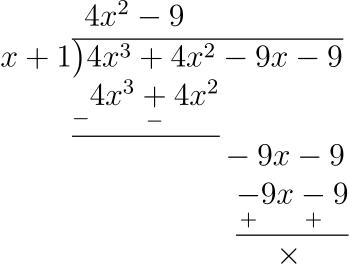we get (4x2 - 9) as quotient and remainder = 0.

$\therefore 4x^3 + 4x^2 - 9x - 9 = (x + 1)(4x^2 - 9) \\[0.5em] = (x + 1)((2x)^2 - (3)^2) \\[0.5em] = (x + 1)(2x - 3)(2x + 3)$

Hence, 4x3 + 4x2 - 9x - 9 = (x + 1) (2x - 3)(2x + 3).

(ii) f(x) = x3 - 19x - 30

Let x = -2, substituting the value of x in f(x),

$f(-2) = (-2)^3 - 19(-2) - 30 \\[0.5em] = -8 + 38 - 30 \\[0.5em] = 0$

Since, f(-2) = 0 hence, (x + 2) is a factor of x3 - 19x - 30.

On dividing, x3 - 19x - 30 by (x + 2),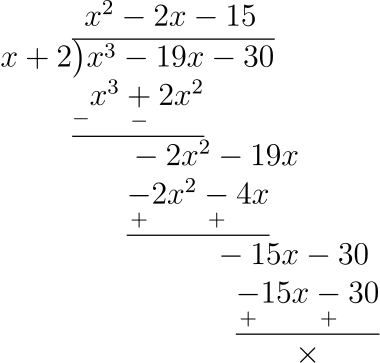we get x2 - 2x - 15 as quotient and remainder = 0.

$\therefore x^3 - 19x - 30 = (x + 2)(x^2 - 2x - 15) \\[0.5em] = (x + 2)(x^2 - 5x + 3x - 15) \\[0.5em] = (x + 2)(x(x - 5) + 3(x - 5)) \\[0.5em] = (x + 2)(x + 3)(x - 5)$

Hence, x3 - 19x - 30 = (x + 2) (x + 3)(x - 5).

#### Question 7

If x3 - 2x2 + px + q has a factor (x + 2) and leaves a remainder 9 when divided by (x + 1), find the values of p and q. With these values of p and q, factorise the given polynomial completely.

By factor theorem (x - b) is a factor of f(x), if f(b) = 0.

f(x) = x3 - 2x2 + px + q

Given, (x + 2) or (x - (-2)) is a factor of f(x).

∴ f(-2) = 0

$\Rightarrow (-2)^3 - 2(-2)^2 + p(-2) + q = 0 \\[0.5em] \Rightarrow -8 - 8 - 2p + q = 0 \\[0.5em] \Rightarrow -2p + q = 16 \\[0.5em] q = 2p + 16 \text{ \space (Equation 1)}$

By remainder theorem, on dividing f(x) by (x - a), the remainder left is f(a).

∴ On dividing f(x) by (x + 1) or (x - (-1)), Remainder = f(-1)

Given, Remainder = 9

∴ f(-1) = 9

$\Rightarrow (-1)^3 - 2(-1)^2 + p(-1) + q = 9 \\[0.5em] \Rightarrow -1 - 2 - p + q = 9 \\[0.5em] \Rightarrow -3 - p + q = 9 \\[0.5em] \Rightarrow q - p = 9 + 3 \\[0.5em] \Rightarrow q - p = 12 \\[0.5em] \text{(Putting value of q = 2p + 16 from equation 1)} \\[0.5em] \Rightarrow 2p + 16 - p = 12 \\[0.5em] \Rightarrow p + 16 = 12 \\[0.5em] \Rightarrow p = 12 - 16 \\[0.5em] \Rightarrow p = -4 \\[0.5em] \text{ and } q = 2p + 16 = 2(-4) + 16 = -8 + 16 = 8$

Now putting p = -4 and q = 8 in f(x),

f(x) = x3 - 2x2 - 4x + 8

Since, (x + 2) is a factor of f(x), on dividing f(x) by (x + 2),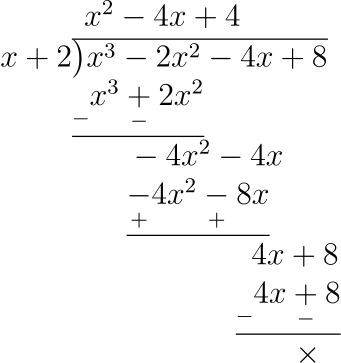we get x2 - 4x + 4 as quotient and remainder = 0.

$\therefore x^3 - 2x^2 - 4x + 8 = (x + 2)(x^2 - 4x + 4) \\[0.5em] =(x + 2)(x^2 - 2 \times 2 \times x + 2^2) \\[0.5em] = (x + 2)(x - 2)^2$

Hence, value of p = -4 and q = 8;
x3 - 2x2 - 4x + 8 = (x + 2) (x - 2)2.

#### Question 8

If (x + 3) and (x - 4) are factors of x3 + ax2 - bx + 24, find the values of a and b. With these values of a and b, factorise the given expression.

By factor theorem (x - b) is a factor of f(x), if f(b) = 0.

f(x) = x3 + ax2 - bx + 24

Given, (x + 3) or (x - (-3) and (x - 4) are factors of f(x)

∴ f(-3) = 0 and f(4) = 0.

For, f(-3) = 0

$\Rightarrow (-3)^3 + a(-3)^2 - b(-3) + 24 = 0 \\[0.5em] \Rightarrow -27 + 9a + 3b + 24 = 0 \\[0.5em] \Rightarrow 9a + 3b - 3 = 0 \\[0.5em] \Rightarrow 9a + 3b = 3 \\[0.5em] \Rightarrow 3a + b = 1 \text{ (On dividing equation by 3) } \\[0.5em] b = 1 - 3a \text{ \space (Equation 1) }$

For f(4) = 0

$\Rightarrow (4)^3 + a(4)^2 - b(4) + 24 = 0 \\[0.5em] \Rightarrow 64 + 16a - 4b + 24 = 0 \\[0.5em] \Rightarrow 16a - 4b + 88 = 0 \\[0.5em] \Rightarrow 16a - 4b = -88 \\[0.5em] \Rightarrow 4a - b = -22 \text{ (On dividing equation by 4) } \\[0.5em] \text{(Putting value of b = 1 - 3a from equation 1) } \\[0.5em] \Rightarrow 4a - 1 + 3a = -22 \\[0.5em] \Rightarrow 7a - 1 = -22 \\[0.5em] \Rightarrow 7a = -22 + 1 \\[0.5em] \Rightarrow 7a = -21 \\[0.5em] \Rightarrow a = -\dfrac{21}{7} \\[0.5em] \Rightarrow a = -3 \\[0.5em] \text{ and } b = 1 - 3a = 1 - 3(-3) = 1 + 9 = 10$

Now putting a = -3 and b = 10 in f(x),

f(x) = x3 - 3x2 - 10x + 24

Since, (x + 3) and (x - 4) is a factor of f(x), hence (x + 3)(x + 4) is also the factor

$(x + 3)(x - 4) = x^2 + 3x - 4x - 12 \\[0.5em] = x^2 - x - 12$

On dividing, f(x) by x2 - x - 12,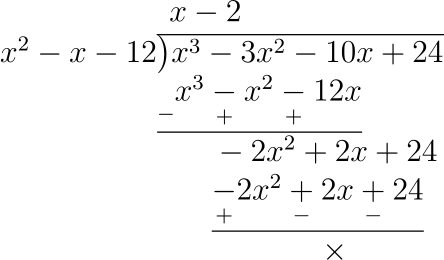we get (x - 2) as quotient and remainder = 0.

$\therefore x^3 - 3x^2 - 10x + 24 = (x - 2)(x^2 - x - 12). \\[0.5em] = (x - 2)(x^2 - 4x + 3x - 12) \\[0.5em] = (x - 2)(x(x - 4) + 3(x - 4)) \\[0.5em] = (x - 2)(x + 3)(x - 4)$

Hence, value of a = -3 and b = 10;
x3 - 3x2 - 10x + 24 = (x - 2)(x + 3)(x - 4).

#### Question 9

If (2x + 1) is a factor of both the expressions 2x2 - 5x + p and 2x2 + 5x + q, find the values of p and q. Hence, find the other factors of both the polynomials.

By factor theorem (x - b) is a factor of f(x), if f(b) = 0.

Let, f(x) = 2x2 - 5x + p

Given, (2x + 1) or 2(x - (-$\dfrac{1}{2}$) is a factor of f(x)

$\therefore f(-\dfrac{1}{2}) = 0 \\[0.5em] \Rightarrow 2\big(-\dfrac{1}{2}\big)^2 - 5\big(-\dfrac{1}{2}\big) + p = 0 \\[0.5em] \Rightarrow 2\big(\dfrac{1}{4}) + \dfrac{5}{2} + p = 0 \\[0.5em] \Rightarrow \dfrac{1}{2} + \dfrac{5}{2} + p = 0 \\[0.5em] \Rightarrow \dfrac{6}{2} + p = 0 \\[0.5em] \Rightarrow 3 + p = 0 \\[0.5em] p = -3$

Putting value of p in f(x)

$f(x) = 2x^2 - 5x - 3 \\[0.5em] = 2x^2 - 6x + x - 3 \\[0.5em] = 2x(x - 3) + 1(x - 3) \\[0.5em] = (2x + 1)(x - 3)$

Hence, p = -3 and other factor is (x - 3).

Let, g(x) = 2x2 + 5x + q

Given, (2x + 1) or 2(x - (-$\dfrac{1}{2}$) is a factor of g(x)

$\therefore g(-\dfrac{1}{2}) = 0 \\[0.5em] \Rightarrow 2\big(-\dfrac{1}{2}\big)^2 + 5\big(-\dfrac{1}{2}\big) + q = 0 \\[0.5em] \Rightarrow 2\big(\dfrac{1}{4}) - \dfrac{5}{2} + q = 0 \\[0.5em] \Rightarrow \dfrac{1}{2} - \dfrac{5}{2} + q = 0 \\[0.5em] \Rightarrow -\dfrac{4}{2} + q = 0 \\[0.5em] \Rightarrow -2 + q = 0 \\[0.5em] q = 2$

Putting value of q in g(x)

$f(x) = 2x^2 + 5x + 2 \\[0.5em] = 2x^2 + 4x + x + 2 \\[0.5em] = 2x(x + 2) + 1(x + 2) \\[0.5em] = (2x + 1)(x + 2)$

Hence, q = 2 and other factor is (x + 2).

#### Question 10

If a polynomial f(x) = x4 - 2x3 + 3x2 - ax - b leaves remainders 5 and 19 when divided by (x - 1) and (x + 1) respectively, find the values of a and b. Hence, determine the remainder when f(x) is divided by (x - 2).

By remainder theorem, on dividing f(x) by (x - a), the remainder left is f(a).

f(x) = x4 - 2x3 + 3x2 - ax - b

∴ On dividing f(x) by (x + 1) or (x - (-1)), Remainder = f(-1)

Given, on dividing by (x + 1) remainder = 19,

∴ f(-1) = 19

$\Rightarrow (-1)^4 - 2(-1)^3 + 3(-1)^2 - a(-1) - b = 19 \\[0.5em] \Rightarrow 1 + 2 + 3 + a - b = 19 \\[0.5em] \Rightarrow a - b + 6 = 19 \\[0.5em] \Rightarrow a - b = 13 \\[0.5em] a = 13 + b \text{ \space (Equation 1) }$

∴ On dividing f(x) by (x - 1), Remainder = f(1)

Given, on dividing by (x - 1) remainder = 5,

∴ f(1) = 5

$\Rightarrow (1)^4 - 2(1)^3 + 3(1)^2 - a(1) - b = 5 \\[0.5em] \Rightarrow 1 - 2 + 3 - a - b = 5 \\[0.5em] \Rightarrow 2 - a - b = 5 \\[0.5em] \Rightarrow -a - b = 3 \\[0.5em] \text{ (Putting value of a = 13 + b from equation 1) } \\[0.5em] \Rightarrow -(13 + b) - b = 3 \\[0.5em] \Rightarrow -13 - b - b = 3 \\[0.5em] \Rightarrow -13 - 2b = 3 \\[0.5em] \Rightarrow 2b = -16 \\[0.5em] \Rightarrow b = -\dfrac{16}{2} \\[0.5em] \Rightarrow b = -8 \\[0.5em] \text{ and } a = 13 + b = 13 - 8 = 5$